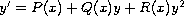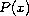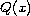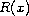Electron. J. Diff. Equ., Vol. 2010(2010), No. 66, pp. 1-10.

### Special solutions of the Riccati equation with applications to the Gross-Pitaevskii nonlinear PDE Anas Al Bastami, Milivoj R. Belic, Nikola Z. Petrovic

Abstract:
A method for finding solutions of the Riccati differential equationis introduced. Provided that certain relations exist between the coefficient,and, the above equation can be solved in closed form. We determine the required relations and find the general solutions to the aforementioned equation. The method is then applied to the Riccati equation arising in the solution of the multidimensional Gross-Pitaevskii equation of Bose-Einstein condensates by the F-expansion and the balance principle techniques.

Submitted April 6, 2010. Published May 8, 2010.
Math Subject Classifications: 34A34, 34A05.
Key Words: Riccati equation; solutions to Gross-Pitaevskii equation; chirp function.

Show me the PDF file (370 KB), TEX file, and other files for this article.Anas Al Bastami Science Program, Texas A&M University at Qatar, P.O. Box 23874 Doha, Qatar email: anas.al_bastami@qatar.tamu.edu Milivoj R. Belic Science Program, Texas A&M University at Qatar, P.O. Box 23874 Doha, Qatar email: milivoj.belic@qatar.tamu.edu Nikola Z. Petrovic Science Program, Texas A&M University at Qatar, P.O. Box 23874 Doha, Qatar email: nikola.petrovic@qatar.tamu.edu